SSC BOARD PAPERS IMPORTANT TOPICS COVERED FOR BOARD EXAM 2024

### MATHS DICTIONARY

Addends: A number that is added; for example, in the expression 11 + 34, 11 and 34 are the addends.
Analog Clock – a clock with the numbers 1 to 12 around the face and rotating hands to show the hours, minutes and seconds.

# Arrays: a set of objects or numbers arranged in order, often in rows or columns.

Arrays can make counting and calculating easier.
ΔΔΔΔΔΔΔ
ΔΔΔΔΔΔΔ

Base (base): the bottom of something, e.g. the bottom line of a plane shape, or the bottom face of a solid.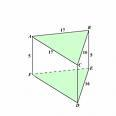# Columns

Compose :
Cubes : Part of base ten material used to represent usually thousands.
Cylinder  : a solid shape with one curved surface and two congruent circular
or elliptical bases.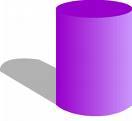Decimals  – A numeral that shows a fractional amount by using digits on either side of a dot called the decimal point; for example, 0.7 is decimal for 7/10 and 2.25 is a decimal for 9/4 or 2 ¼.
Decimetre (dm) – a unit used to measure length (1 dm = 10 cm)
Decompose  -
Denominator – The number below the line in a fraction; the denominator tells the number of parts into which the whole has been divided.
Difference - The difference of two numbers is the result of subtraction. For example, subtracting 3 from 5 gives the difference of 2, written
5 – 3 = 2.
Digital Clock: a clock that uses numerals to show the time.
Digits  : Any of the symbols 0, 1, 2, 3, 4, 5, 6, 7, 8, 9 used to write numbers.
Distance: the length between two points ( or objects.)
Edges: where two surfaces join (intersect).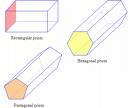# Equal shares:

Equation : A mathematical statement that two expressions are equal; for example, 2 + x = 14.
Equivalent factions – Fractions that have the same value; for example 2/3 and 6/9
Estimate: close to an amount or value but not exact.
Faces: flat surfaces of a three-dimensional shape.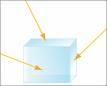Facts: The basic operations of math, like addition, subtraction, and multiplication and division.
Fact family: Fact families are 3 numbers that are related, just as the people in a family are related. They make a set of related math facts.
Add and subtract with facts 3, 5, 8:
3 + 5 = 8
5 + 3 = 8
8 – 3 = 5
8 – 5 = 3

Multiply and divide with facts 3, 5, 15:
3 x 5 = 15
5 x 3 = 15
15 ÷ 3 = 5
15 ÷ 5 = 3

# Factors:  A whole number that divides exactly into another whole number.

A whole number that multiplies with another whole number to make a third number.
Numbers that are multiplied to get a product.

12 = 1 x 12 12 /1 = 12
12 = 3 x 4 12 / 3 = 4
12 = 2 x 6 12/6  = 2

Flats  : Part of base ten material used to represent usually hundreds.
Greater than : More than; shows relationship between numbers;
symbol (>); example:  10 > 9 ( 10 is greater than 9 )

# Grouping: Dividing things into equal groups (sets.)

Half - The irreducible faction resulting from dividing one by two (1/2), or any number by it double.  It is the faction occurring most often in mathematical equations, recipes, measurements etc.
Hexagon  – A six-sided polygon.
Hour  : 60 minutes
Hundreds  : Each digit of a number in our number system has a different place value (ones, tens, hundreds, etc.). In the example, the underlined digit is in the hundreds position.
Example: 1783  ( 7 is in the hundreds position and has a value of 700)
Intersecting Lines: lines that cross over one another; or overlap.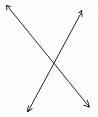Length:  distance from one end to the other; how long something is.

Less than: Less than; shows relationship between numbers;
symbol (<); example:  5 < 9 ( 5 is less than 9 )

Metre (m)  – a unit used to measure length  ex: 1 m =  100 cm,
1000 m = 1 km

Minute: 60 seconds.

Mirror Image: a mirror-based duplicate of a single image.

# Multiples : Mathematic operation where a number is added to itself a number of times.

Eg. 4 + 4 + 4 + 4 + 4 = 20
or  4 x 5 = 20

Nets/patterns  flat shape which can be folded up into a three-dimensional
solid.
Number family: Number Family is a given number, its partners (2 numbers that add up to the number), and all of the addition and subtraction facts that can be generated from the partners. A child working on the Number Family of 8 would be studying and learning the partners and all of the corresponding addition and subtraction facts for 8.
Example: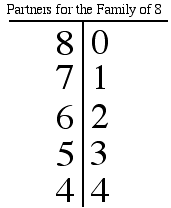Math facts for the number family of 8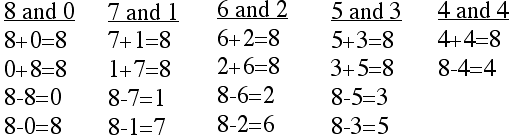Number line – line marked with numbers, used to show operations.
- has numbers in order from least to greatest. The spaces between pairs of consecutive numbers are equal.Number sentence: An arrangement of numbers and symbols; for example, an addition sentence: 3 + 7 = 10; a subtraction sentence: 12 – 4 = 8; a multiplication sentence: 5 x 7 = 35; a division sentence: 48 ÷ 6 = 8
Numerator – The number above the line in a fraction; the numerator tells how many parts are being considered.
Octagon – A polygon with 8 sides.
Ordering : Arranging according to size, amount or value.

Parallel lines: Lines that are the same distance apart.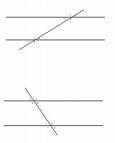Patterns: repeated design or recurring sequence.

Perpendicular Lines : all right angles to the horizon.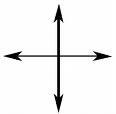# or 5 x 2 = 10.

Pyramid:  a solid shape with a polygon as a base and triangular faces that
taper to a point (vertex).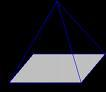Quadrilateral – A figure with 4 sides.
Quarter/Fourth  -
Quotient  – The number obtained by dividing one number into another.  In the division sentence:
77 div 11 = 7, the quotient is 7
Part :
Pentagon  – A five-sided polygon.

Rectangle – A quadrilateral, where 2 pairs of opposite sides are equal and each angle is a right angle, or 90 degrees.
Rectangular Prism:  a polyhedron-a prism with two identical, rectangular bases.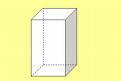Reflective Symmetry: Line symmetry means reflection. If we were to draw a line through a shape to represent a mirror, the shape could be folded along that line and both sides would both fit exactly together.
Regroup: rearrange the formation of a group; used to assist when trading or carrying in addition or subtraction.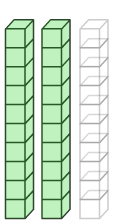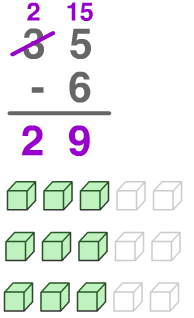Remainder – What is left over when one number does not divide exactly into another number.  For example, in the quotient 13 divided 5 = 2RC, the remained is 3.

Rhombus – A parallelogram with four equal sides.
Rods  : Part of base ten material used to represent usually tens.
Round: change a number to a more convenient value.

# Rows :  Items arranged in a horizontal line.

Space: the three dimensional place in which an object can exist or events take place.
Square  – A quadrilateral with equal sides and each angle is a right angle, or 90 degrees.
Sum  - A sum is the result of an addition. For example, adding 1, 2, 3, and 4 gives the sum 10, written
 1 + 2+ 3 + 4 = 10 Tenths  – The place value representing 0.1, or 1/10; for example, three tenths is 0.3, or 3/10.
Thousands:  Each digit of a number in our number system has a different place value (ones, tens, hundreds, etc.). In the example, the underlined digit is in the hundreds position.
Example: 2783  ( 2 is in the thousands position and has a value of 2000)

# Trains/towers –

Trapezoid – A quadrilateral where 1 pair of sides is parallel.

Triangular Prism: a polyhedron-a prism with two identical, triangular
bases.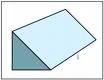Units : Part of base ten material used to represent usually ones.
Value: numerical worth or amount.
Vertex/vertices: point where surfaces meet-corner.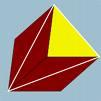Width: distance across from side to side.

Whole  – Any of the natural numbers (positive or negative) or Zero.  The whole numbers are the counting numbers and 0.  The whole numbers are 0, 1, 2, 3, 4, 5…